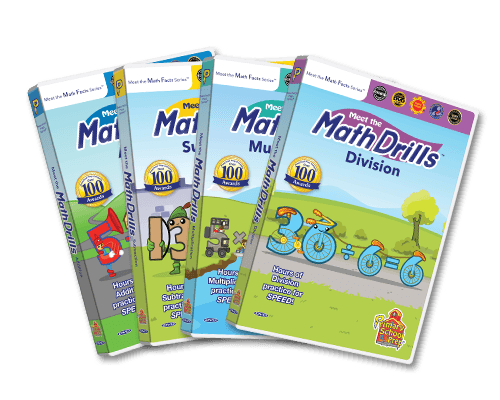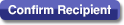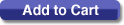# Meet the Math Drills 4 Pack (DVDs)Purchase options:Start Over?Math Drills Explained

Practicing Addition Has Never Been This Much Fun!

Meet the Math Facts DVDs made learning math facts fun and easy. Now it is time to practice what you have learned. Meet the Math Drills Addition provides hours of practice so that you will be able to recall addition equations to 10+10 with rapid SPEED!

Meet the Math Drills: Subtraction

Practicing Subtraction Has Never Been This Much Fun!

Meet the Math Facts DVDs made learning math facts fun and easy. Now it is time to practice what you have learned. Meet the Math Drills Subtraction provides hours of practice so that you will be able to recall subtraction equations from 0 to 10 with rapid SPEED!

Meet the Math Drills: Multiplication

Practicing Multiplication Has Never Been This Much Fun!

Meet the Math Facts DVDs made learning math facts fun and easy. Now it is time to practice what you have learned. Meet the Math Drills Multiplication provides hours of practice so that you will be able to recall multiplication equations to 12�12 with rapid SPEED!

Meet the Math Drills: Division

Practicing Division Has Never Been This Much Fun!

Meet the Math Facts DVDs made learning math facts fun and easy. Now it is time to practice what you have learned. Meet the Math Drills Division provides hours of practice so that you will be able to recall division equations from 0 to 12 with rapid SPEED!